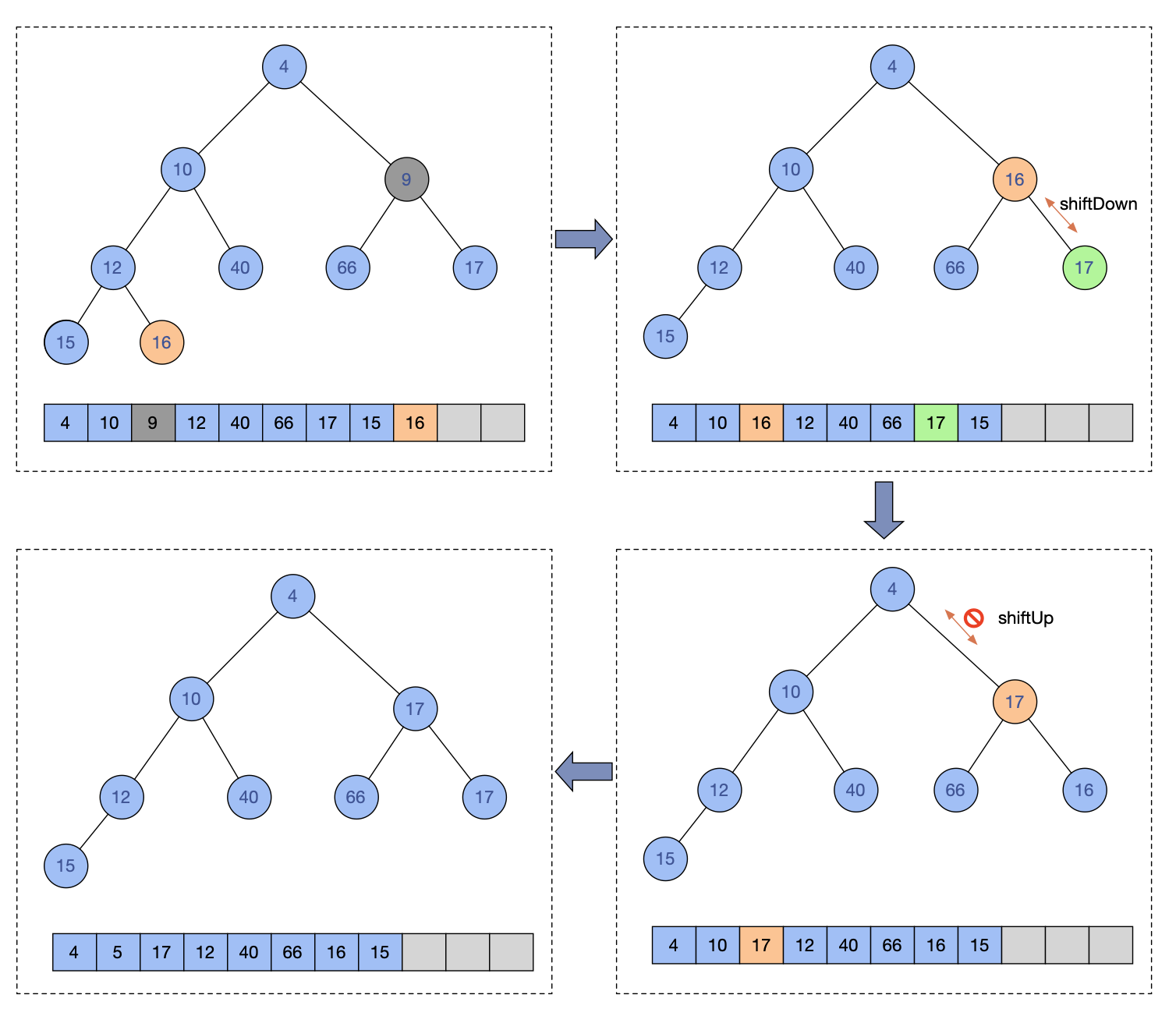## JDK源码分析实战系列-PriorityQueue

### 堆

• 堆中某个结点的值总是不大于或不小于其父结点的值。
• 堆总是一棵完全二叉树。

### PriorityQueue

Priority queue represented as a balanced binary heap: the two children of queue[n] are queue[2n+1] and queue[2(n+1)]

The element with the lowest value is in queue, assuming the queue is nonempty

PriorityQueue就是一个小顶堆的实现，也是被认为实现优先级队列最高效的方式。

#### 插入元素操作

public boolean offer(E e) {
if (e == null)
throw new NullPointerException();
modCount++;
int i = size;
if (i >= queue.length)
// 扩容
grow(i + 1);
// 最后一个位置下标加1
size = i + 1;
if (i == 0)
// 第一个元素情况
queue = e;
else
// 节点从尾部加入，然后上移操作，直到保持堆处于正确状态
siftUp(i, e);
return true;
}


PriorityQueue支持元素实现Comparable，也支持初始化时传入一个Comparator作为比较算子。所以siftUp区分了两种实现，都是差不多的，选一个分析一下，siftUp是调整堆的核心操作，这个操作是把元素从参数k位置开始，和父节点进行比较，如果比父节点小，就和父节点交换，不断重复，直到父节点比自己大或等于，或者自己已经移动到根节点才停止。

private void siftUp(int k, E x) {
if (comparator != null)
siftUpUsingComparator(k, x);
else
siftUpComparable(k, x);
}
private void siftUpComparable(int k, E x) {
Comparable<? super E> key = (Comparable<? super E>) x;
while (k > 0) {
// 父节点下标
int parent = (k - 1) >>> 1;
// 父节点值
Object e = queue[parent];
// 新增值大于父节点，那就符合成为这个父节点下子节点的要求
if (key.compareTo((E) e) >= 0)
break;
// 当前的父节点下移动
queue[k] = e;
// k改为父节点下标，下一轮循环从父节点开始
k = parent;
}
// 退出循环出来的，直接把值赋值给k下标即可
queue[k] = key;
}#### 查询元素

public boolean contains(Object o) {
return indexOf(o) != -1;
}
private int indexOf(Object o) {
if (o != null) {
// 遍历数组
for (int i = 0; i < size; i++)
// 比较查出想要找的元素
if (o.equals(queue[i]))
return i;
}
// 如果不存在就返回-1 contains判断是否不等于-1
return -1;
}


#### 删除元素操作

public boolean remove(Object o) {
int i = indexOf(o);
if (i == -1)
return false;
else {
removeAt(i);
return true;
}
}
private E removeAt(int i) {
// assert i >= 0 && i < size;
modCount++;
// 数组最后一个元素的下标
int s = --size;
if (s == i) // removed last element
queue[i] = null;
else {
// 数组最后一个元素的值
E moved = (E) queue[s];
// 将数组最后一个元素从位置上删除
queue[s] = null;
// 将元素从要删除的数组下标i位置开始下移
// 下移只能在i下标是非叶子节点情况，并且子节点大于移动的值才会发生下移
siftDown(i, moved);
// 这个判断表示如果i下标已经是叶子节点而没有下移，或者i下标不是叶子节点在和子节点比较后而无需下移
if (queue[i] == moved) {
// 既然没有进行下移，那么说明在i这个位置上，放moved这个值是最小的，但是还不能保证和自己的父节点比是不是大于等于的，所以需要进行上移的操作
siftUp(i, moved);
// 上移结束后，如果i下标的元素和moved不一致证明在这个i下标之前的元素已经因为上移操作有了变化，而返回这个从数组最后取得的元素
if (queue[i] != moved)
return moved;
}
}
return null;
}


siftUp相似，siftDown操作也是区分两种方式，这里也只挑一个看就行了。

private void siftDown(int k, E x) {
if (comparator != null)
siftDownUsingComparator(k, x);
else
siftDownComparable(k, x);
}
private void siftDownComparable(int k, E x) {
Comparable<? super E> key = (Comparable<? super E>)x;
// 这个half是最后一个节点无符号右移计算得到
// 这个计算得到的位置是叶子节点的开始位置，所以下面的while条件是小于这个位置
int half = size >>> 1;        // loop while a non-leaf
// 因为这里是操作下移，如果已经是叶子节点就没有下移的必要了
while (k < half) {
// 自己左侧子节点下标
int child = (k << 1) + 1; // assume left child is least
// 自己左侧子节点值
Object c = queue[child];
// 自己右侧子节点下标
int right = child + 1;
// 右节点可能没有，比如左节点已经是数组最后一个值了，所以判断了一下right < size
if (right < size &&
((Comparable<? super E>) c).compareTo((E) queue[right]) > 0)
// 如果如果左节点值大于右节点值，就把更小的右节点值赋值给c
c = queue[child = right];
if (key.compareTo((E) c) <= 0)
// 如果自己比左节点值小，说明不能下移了，就退出循环
break;
// 执行到这里，说明自己作为父节点，并不比子节点小
// 那么就把前面从左右子节点中找出来最小的值赋值给k下标的位置
queue[k] = c;
// k值变更为较小的值的下标
k = child;
}
// 循环退出后，k下标的位置放自己
queue[k] = key;
}


siftDown它的核心逻辑是将一个元素往树的下层进行比较，找到比自己小的就进行交换。注意，这种比较是需要比较自己子节点的左右节点的，毕竟这棵树只保证父节点小于子节点，并不保证左右的节点大小顺序。#### 获取顶部元素

poll是肯定要用的方法，毕竟花了这么大劲把最小值排到了根节点上。

public E poll() {
// 空的情况
if (size == 0)
return null;
// 数组有值的最后一个下标
int s = --size;
modCount++;
// 根节点，返回值
E result = (E) queue;
// 最后一个元素
E x = (E) queue[s];
// 清空根节点
queue[s] = null;
if (s != 0)
// 下移
siftDown(0, x);
return result;
}


#### 扩容

private static final int MAX_ARRAY_SIZE = Integer.MAX_VALUE - 8;
private void grow(int minCapacity) {
int oldCapacity = queue.length;
// Double size if small; else grow by 50%
// 64区分两种扩容数量策略
int newCapacity = oldCapacity + ((oldCapacity < 64) ?
(oldCapacity + 2) :
(oldCapacity >> 1));
// overflow-conscious code
// 虽然是无限优先级队列，最大的容量溢出还是要控制的
if (newCapacity - MAX_ARRAY_SIZE > 0)
newCapacity = hugeCapacity(minCapacity);
queue = Arrays.copyOf(queue, newCapacity);
}
private static int hugeCapacity(int minCapacity) {
// minCapacity 是size+1获得 如果此时size已经是MAX_VALUE 那么minCapacity会变成负数，溢出抛出OutOfMemoryError异常
if (minCapacity < 0) // overflow
throw new OutOfMemoryError();
return (minCapacity > MAX_ARRAY_SIZE) ?
Integer.MAX_VALUE :
MAX_ARRAY_SIZE;
}


#### 关于如何获得顺序数据

public static void main(String[] args) {
PriorityQueue<Integer> priorityQueue = new PriorityQueue<>(10);
for (int i = 10; i >=2; i--) {
}

for (Integer e : priorityQueue) {
System.out.print(e + ",");
}
}


    public static void main(String[] args) {
PriorityQueue<Integer> priorityQueue = new PriorityQueue<>(10);
for (int i = 10; i >=2; i--) {
}

Integer t;
while ((t = priorityQueue.poll()) != null) {
System.out.print(t + ",");
}
}


#### 使用场景

1，高性能定时器 这个在jdk中的ScheduledExecutorService有使用，以后分析可以关联起来。

2，Top K 问题，实际业务研发会使用到。

posted on 2022-11-20 17:25  每当变幻时  阅读(547)  评论(0编辑  收藏  举报